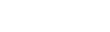# In this article, I will go over a popular homogenous model ensemble method — bagging. Homogenous ensembles combine a large number of base estimators or weak learners of the same algorithm.Claudia

4 months ago | 4 min read
Follow

## Understand the intuition behind bagging with examples in Python

In this article, I will go over a popular homogenous model ensemble method — bagging. Homogenous ensembles combine a large number of base estimators or weak learners of the same algorithm.

The principle behind homogenous ensembles is the idea of “wisdom of the crowd” — the collective predictions of many diverse models is better than any set of predictions made by a single model. There are three requirements to achieve this:

1. The models must be independent;
2. Each model performs slightly better than random guessing;
3. All individual models have similar performance on their own.

When these three requirements are satisfied, adding more models should improve the performance of your ensemble.

Ensemble methods help to reduce variance and combat overfitting to your train dataset, thus allowing your model to better learn generalized patterns rather than overfitting to the noise in your train dataset.

# Bagging

## How Bagging Works

In bagging, a large number of independent weak models are combined to learn the same task with the same goal. The term “bagging” comes from bootstrap + aggregating, whereby each weak learner is trained on a random subsample of data sampled with replacement (bootstrapping), and then the models’ predictions are aggregated.

Bootstrapping guarantees independence and diversity, because each subsample of data is sampled separately with replacement and we are left with different subsets to train our base estimators.

The base estimators are weak learners that perform only slightly better than random guessing. An example of such a model is a shallow decision tree limited to a maximum depth of three. The predictions from these models are then combined through averaging.

Bagging can be applied to both classification and regression problems. For regression problems, the final predictions will be an average (soft voting) of the predictions from base estimators. For classification problems, the final predictions will be the majority vote (hard voting).

Diagram of Bagging Algorithms

## Implementing Bagging Algorithms with Scikit-Learn

You can build your own bagging algorithm using BaggingRegressor or BaggingClassifier in the Python package Scikit-Learn.

To begin, instantiate your base estimator and enter this as your base estimator in BaggingRegressor or BaggingClassifier. Below are an example of a bagging regressor with a linear regression as the base estimator, and an example of a bagging classifier with a decision tree classifier as the base estimator. The default number of estimators is 10.

``from sklearn.linear_model import LinearRegressionfrom sklearn.ensemble import BaggingRegressorreg_lr = LinearRegression()reg_bag = BaggingRegressor(base_estimator=reg_lr)reg_bag.fit(X_train, y_train)====================================================================from sklearn.tree import DecisionTreeClassifierfrom sklearn.ensemble import BaggingClassifierclf_dt = DecisionTreeClassifier(max_depth=3)clf_bag = BaggingClassifier(base_estimator=clf_dt)clf_bag.fit(X_train, y_train)``

## Random Forest

Random forest is a popular example of a bagging algorithm. It uses averaging to ensemble a number of individual decision trees trained on a subset of the train dataset.

Using scikit-learn’s random forest in Python, you can specify tree-specific parameters. The following are some important hyperparameters to tune so that it is optimized for your dataset:

• n_estimators: number of trees to train to be aggregated. Usually between 100 to 500 trees is enough and generally, more trees will improve your model (with diminishing returns) but it will also be more computationally expensive;
• max_depth : the maximum depth of a tree. A deeper tree will help to reduce the bias but at the expense of increasing variance. The aggregation of multiple trees in random forest can help to combat this, but you should still be careful;
• max_features: the maximum number of features to consider at each split. A good starting point is usually the square root of the number of features.

Another important concept in random forest is the out-of-bag (OOB) score. When performing bootstrapping, there will be instances left out of the subsamples used to train the estimators.

These out-of-sample instances can be used to evaluate the model to obtain an out-of-bag (OOB) score, in essence like a pseudo-validation set for the random forest model. To obtain the OOB score, set oob_score=True when initializing your random forest object.

``rf = RandomForestClassifier(n_estimators=100, oob_score=True)rf.fit(X_train, y_train)print(rf.oob_score_)``

Note that scikit-learn’s random forest algorithms will return an error if there are null values in your features, so remember to fill null values with pandas’ fillna before calling fit on your data, otherwise it will throw an error.

## Pros and Cons of Bagging

• Reduces variance: Given that the sampling is done truly randomly with bootstrapping, bagging usually helps to reduce variance and combat overfitting.
• Easy to parallelize: Estimators are independent, so models can be built in parallel with bagging.
• Greater stability and robustness: The high number of estimators aggregated together help to provide more stability and robustness to the predictions.
• Difficult to interpret: The final predictions of a bagging algorithm are based on the mean predictions from the base estimators. While this improves accuracy, it becomes more difficult to interpret the model.

# Summary

Bagging is based on the idea of collective learning, where many independent weak learners are trained on bootstrapped subsamples of data and then aggregated via averaging. It can be applied to both classification and regression problems.

Random forest is a popular example of a bagging algorithm. When tuning hyperparameters in random forest for your dataset, the important three areas to pay attention to are: i) number of trees (n_estimators), ii) prune the trees (start with max_depth but also explore samples required in a node and/or for splitting), iii) the maximum number of features to consider at each split (max_features).

Bagging is a very powerful concept and example of model ensemble methods. Hope this inspires you to try out a bagging algorithm next time you approach a predictive modeling problem!

Originally published here!

UpvoteCreated by

Claudia

Follow

Data SciencePost

Upvote

Downvote

Comment

Bookmark

Share

Related Articles